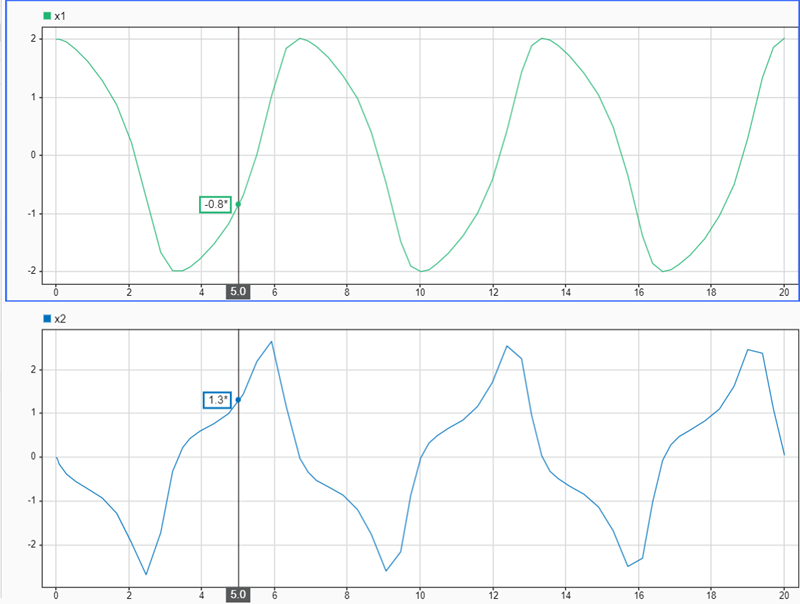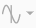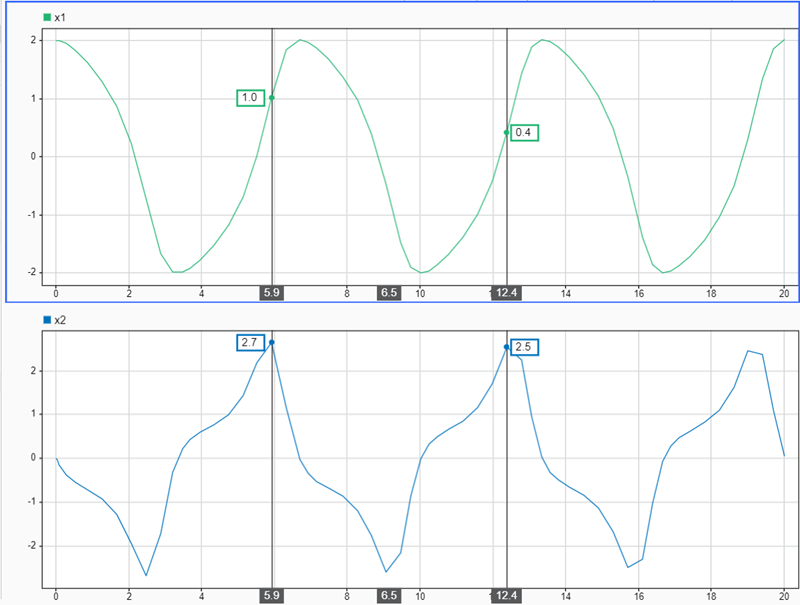Check number of cursors active in the Simulation Data Inspector

## Syntax

``num = Simulink.sdi.getNumCursors``
``num = Simulink.sdi.getNumCursors(view)``

## Description

````num = Simulink.sdi.getNumCursors` returns the number of active cursors in the Inspect pane of the Simulation Data Inspector.```

example

````num = Simulink.sdi.getNumCursors(view)` returns the number of active cursors in the Inspect or Compare pane of the Simulation Data Inspector, as specified by `view`.```

## Examples

collapse all

You can use the Simulation Data Inspector programmatic interface to specify the position of cursors on time plots or sparklines and to access the time that corresponds to the current cursor position.

Open the session file `vdp_mu.mldatx` to add plot data in the Simulation Data Inspector. The session file contains data for a 20-second simulation of a model of the Van Der Pol equation, with signals `x1` and `x2` plotted on separate subplots in a `2`-by-`1` subplot layout.

`open vdp_mu.mldatx;`

Use the `Simulink.sdi.setNumCursors` function to add one cursor to the plot.

`Simulink.sdi.setNumCursors(1);`

Suppose you want to know the signal values `5` seconds into the simulation. Use the `Simulink.sdi.setCursorPositions` function to move the cursor to `t=5s` and read the signal values off from the cursor on each subplot. The asterisk next to the value in the cursor label indicates that the value is interpolated because the simulation did not include a calculation for a simulation time of exactly `5s`.

`Simulink.sdi.setCursorPositions('left',5);`Suppose you want to calculate the time between two peaks in the `x2` signal. Add a second cursor using the `Simulink.sdi.setNumCursors` function or by using Show/hide data cursorsin the Simulation Data Inspector.

```numCursors = Simulink.sdi.getNumCursors; if(numCursors < 2) Simulink.sdi.setNumCursors(2); end```

Drag the cursors so the left cursor is positioned at the first peak in the `x2` signal and the right cursor is positioned at the second peak. Alternatively, you can run this code that positions the cursors for you.

```Simulink.sdi.setCursorPositions('left',5.921998549931304,... 'right',12.378442136906246);```The Simulation Data Inspector displays the difference between the two cursors in seconds. However, the cursor position and difference values are both rounded. Use the `Simulink.sdi.getCursorPositions` function to programmatically retrieve the exact time values that correspond to the cursor positions. Then, compute the difference between the times.

```[t1,t2] = Simulink.sdi.getCursorPositions; T = t2 - t1```
`T = 6.4564`

## Input Arguments

collapse all

Plot area for which to return number of active cursors, specified as `'inspect'` or `'compare'`.

Data Types: `char` | `string`

## Output Arguments

collapse all

Number of active cursors, returned as `0`, `1`, or `2`.

## Version History

Introduced in R2021a